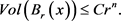# Ricci孤立子的刚性及体积增长Rigidity and Volume Growth of Ricci Solitons

DOI: 10.12677/PM.2019.91002, PDF, HTML, XML, 下载: 612  浏览: 2,298  科研立项经费支持

Abstract: After the concept of Ricci flow was proposed by Hamilton in the 1980s and applied to solve the Poincaré conjecture, the classification and geometry of the self-similar solution of Ricci flow (i.e., Ricci soliton) developed rapidly. A gradient soliton is said to be rigid if it is isometric to a quotient of N×Rk, where N is an Einstein manifold. Volume growth of geodesic balls is an important geometric property for studying manifolds and Ricci soliton and the volume growth rate is an im-portant geometric invariant of manifolds. In this paper, we will systematically summarize the basic development of Ricci solitons, the rigidity and volume growth results of Ricci solitons, and give the conclusion that the complete non-compact gradient Ricci solitons have linear or Euclidean volume growth.×

1. 引言

1.1. Ricci孤立子介绍

${R}_{ij}+\frac{1}{2}\left({\nabla }_{i}{V}_{j}+{\nabla }_{j}{V}_{i}\right)=\rho {g}_{ij},$

$\rho$ 为常数。此外，若 $V$ 为一个梯度向量场，使得光滑函数 $f$ 满足

${R}_{ij}+{\nabla }_{i}{\nabla }_{j}f=\rho {g}_{ij}.$

$Ric+{\nabla }^{2}f=\rho g$$\rho =0$ 时称为稳定Ricci孤立子， $\rho >0$ 为收缩Ricci孤立子， $\rho <0$ 时称为扩张Ricci孤立子。

1.2. 典型的Ricci孤立子

1) $\left({R}^{n},{g}_{0},\frac{{|x|}^{2}}{4}\right)$ 为带有势函数 $f=\frac{{|x|}^{2}}{4}$ 的梯度收缩孤立子，且 $Ric+{\nabla }^{2}f=\frac{1}{2}{g}_{0}$

2) $\left({R}^{n},{g}_{0},-\frac{{|x|}^{2}}{4}\right)$ 为势函数是 $f=-\frac{{|x|}^{2}}{4}$ 的梯度扩张孤立子，且 $Ric+{\nabla }^{2}f=-\frac{1}{2}{g}_{0}$

2. Ricci孤立子的刚性

Hamilton  (2维情形)及Ivey  (3维情形)证明了紧致Ricci孤立子为刚性的。在Ni-Wallach  工作的基础上，Naber证明了任意具有非负曲率算子的4维完备收缩Ricci孤立子是刚性的，著名的Bryant Ricci孤立子表明存在具有正曲率算子的非刚性旋转对称的稳定及扩张梯度Ricci孤立子。此外不难看出，任意维紧致稳定及扩张梯度Ricci孤立子是刚性的。紧致流形其数量曲率为常数时为刚性的，更一般的，Petersen-Wylie  证明了以下定理：

3. 体积增长估计

Hamilton  系统地开发了Ricci流降低维数的研究方法。由Hamilton对2维Ricci流古典解的分类可知任意具有有界曲率的2维完备古典解都不能达到最大体积增长。在此基础上应用降维方法，Perelman  证明了：

$\underset{r\to +\infty }{\mathrm{lim}}\frac{Vo{l}_{t}\left({B}_{t}\left({x}_{0},r\right)\right)}{{r}^{n}}=0.$

$\underset{r\to \infty }{\mathrm{lim}}\frac{Vol\left({B}_{{x}_{0}}\left(r\right)\right)}{{r}^{n}}=0.$

$Vol\left({B}_{{x}_{0}}\left(r\right)\right)\le C{r}^{n}.$

$Vol\left({B}_{{x}_{0}}\left(r\right)\right)\le C{\left(r+1\right)}^{n-\left(2-\eta \right)\delta }.$

$-r\le f\left(y\right)-f\left(x\right)\le -r+C\mathrm{ln}r.$

$Vol\left(B\left(x,r\right)\right)\le C{r}^{n}.$

$Vol\left(B\left(x,r\right)\right)\le C{r}^{n-\delta }.$

${R}_{ij}+{\nabla }_{i}{\nabla }_{j}f=\frac{1}{2}{g}_{ij}$。则存在常数 $k=k\left({\mu }_{0}\right)>0$，使得当 $r\le 1$$R\le \frac{C}{{r}^{2}}$ 时有：

$Vol\left({B}_{r}\right)\ge k{r}^{n}.$

$Vol\left({B}_{{x}_{0}}\left(r\right)\right)\ge C{r}^{n-2\delta }.$

$Vol\left({B}_{{x}_{0}}\left(r\right)\right)\ge C\mathrm{ln}\mathrm{ln}r.$

$Vol\left({B}_{{x}_{0}}\left(r\right)\right)\ge Cr.$

$\frac{Vol\left({B}_{s}\right)}{{s}^{n}}\le C\cdot {e}^{\frac{-1}{a}{s}^{a}}.$

$\underset{s\to \infty }{\mathrm{lim}}\frac{Vol\left({B}_{s}\right)}{{s}^{n}}\ge \eta .$

$Vol\left({B}_{r}\left(x\right)\right)\ge C{r}^{n}.$$c\cdot r\le Vol\left({B}_{{x}_{0}}\left(r\right)\right)\le c{e}^{a\sqrt{r}}.$

NOTES

*通讯作者。